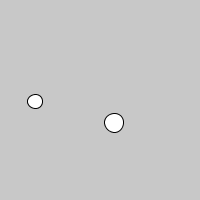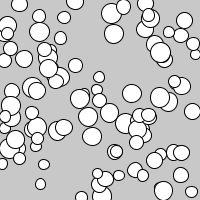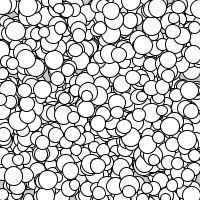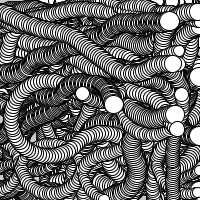# Flocking

example processing creating-classes animation flocking emergence

This program uses a `Flocker` class to encapsulate all of the logic for creating a flocking simulation. Each individual `Flocker` follows simple rules: it finds the closest other `Flocker`, and then turns towards it.

To understand this better, let’s start out with only two `Flocker` instances:

``````Flocker[] flock = new Flocker;

void setup() {
size(200, 200);

for (int i = 0; i < flock.length; i++) {
flock[i] = new Flocker();
}
}

void draw() {

background(200);

for (int i = 0; i < flock.length; i++) {
flock[i].step();
flock[i].draw();
}
}

class Flocker {

float x = random(width);
float y = random(height);
float speed = random(1, 3);

void step() {

//find the closest Flocker
float closestDistance = 100000;
Flocker closestFlocker = null;
for (int i = 0; i < flock.length; i++) {

//make sure not to check against yourself
if (flock[i] != this) {
float distance = dist(x, y, flock[i].x, flock[i].y);
if (distance < closestDistance) {
closestDistance = distance;
closestFlocker = flock[i];
}
}
}

float angleToClosest = atan2(closestFlocker.y-y, closestFlocker.x-x);

//prevent case where heading is 350 and angleToClosest is 10
angleToClosest += TWO_PI;
} else if (angleToClosest-heading > PI) {
angleToClosest -= TWO_PI;
}

//turn towards closest
} else {
}

//move in direction

//wrap around edges
if (x < 0) {
x = width;
}
if (x > width) {
x = 0;
}

if (y < 0) {
y = height;
}
if (y > height) {
y = 0;
}
}

void draw() {
}
}
``````Watch each `Flocker` and notice that it’s just continuously trying to follow the other one.

Code Editor

See the Pen by Happy Coding (@KevinWorkman) on CodePen.

Since we’re using an array, we can easily change the program to use 100 `Flocker` instances:Or even 1000:Even though each individual `Flocker` is only following simple rules, the program itself is a basic simulation of how birds flock, or how bugs swarm, or how a school of fish swims. This idea of complicated (or even beautiful) patterns emerging from simple rules is called emergence!## Tweak Ideas

• The flocking logic here is very simple and just uses cohesion (get close to your neighbors). Better simulations also take separation (don’t get too close to your neighbors) and alignment (fly in the same direction as neighbors) into account. You could also consider the closest 5 (or 10, or…) neighbors instead of only the closest one, or you could consider all of the neighbors within some distance.
• Give each `Flocker` a random color.
• Add a new `Flocker` whenever the user clicks (or drags!) the mouse.
• Move the call to `background()` to the `setup()` function to show trails.
• Make them run away from each other instead of chase each other.
• Make one `Flocker` “it” and have it run away from all of the other `Flockers` while they chase it- or vice-versa!